# Kummer extension

(diff) ← Older revision | Latest revision (diff) | Newer revision → (diff)

An extension of a fieldof characteristic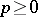, of the type(1)

where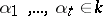,is some natural number, and it is assumed thatcontains a primitive-th root of unity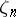(in particular,is prime toif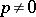). Kummer extensions are named after E. Kummer, who first studied extensions of the type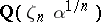, whereis the field of rational numbers and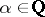.

The main result of the theory of Kummer extensions is that if the fieldcontains a primitive root, then a finite extension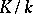is Kummer (for a given) if and only ifis a normal Abelian extension and the Galois groupis annihilated by. Any Kummer extension of a fieldis completely determined by its Kummer group, where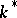is the multiplicative group ofand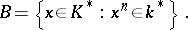There exists a non-degenerate Kummer pairing, i.e. a mapping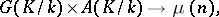where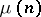is the subgroup ofgenerated by. Ifand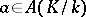, the pairing is defined by the formula, where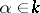, andis some representative of the element. The pairing defines a canonical isomorphism.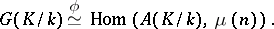(2)

In other words, any automorphism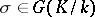is defined by its action on the roots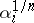in (1), and this action may be arbitrary, provided that the rootsare independent. In particular, ifis a cyclic group, then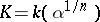, where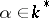.

Letbe a normal extension of a field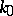and letbe a Kummer extension. Then the fieldis normal overif and only if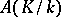is mapped into itself by. In that case the isomorphism (2) is a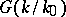-isomorphism, i.e. if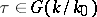,and ifthen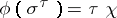, where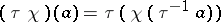. (The groupacts onvia conjugation in.) By the above proposition, many problems concerning Abelian extensions of exponentof a fieldcan be reduced to the theory of Kummer extensions even if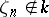. To be precise: Ifis such an extension, then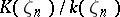is a Kummer extension, and its Kummer group is characterized by the condition: If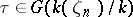and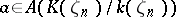, then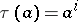, whereis a natural number which is defined moduloby the condition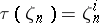.

The main results concerning Kummer extensions may be derived as corollaries of the Hilbert theorem on cyclic extensions, according to which the one-dimensional Galois cohomology group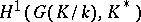is trivial.

The theory of Kummer extensions carries over to the case of infinite Abelian extensions of exponent. When this is done, the Kummer pairing establishes a Pontryagin duality between the profinite group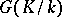(endowed with the Krull topology) and the discrete group(see , ).

The theory of Kummer extensions, also known as Kummer theory, has an analogue in the case of extensions (1) with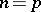(Artin–Schreier theory). The role of the groupin that situation is played by the additive group of the prime subfield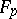of. The main result of the theory is: Any Abelian extensionof exponentof a fieldis of the form, whereare roots of equations of the type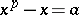(see ). There is also a generalization of this theory, due to E. Witt, to the case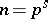, where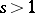, using Witt vectors (cf. Witt vector).

Finally, an attempt has been made to construct a non-Abelian "Kummer theory" , where the multiplicative group of the field is replaced by the matrix group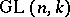.

How to Cite This Entry:
Kummer extension. Encyclopedia of Mathematics. URL: http://encyclopediaofmath.org/index.php?title=Kummer_extension&oldid=15655
This article was adapted from an original article by L.V. Kuz'min (originator), which appeared in Encyclopedia of Mathematics - ISBN 1402006098. See original article